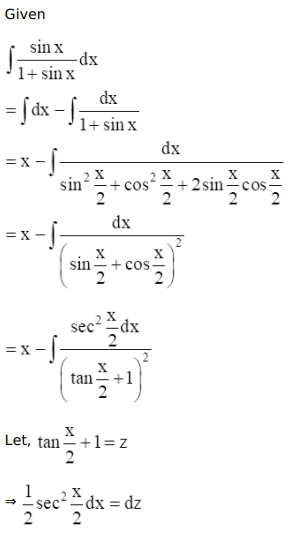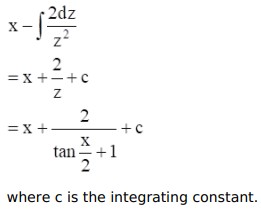# Mark against the correct answer in each of the following:

Question:

Mark $(\sqrt{)}$ against the correct answer in each of the following:

$\int \frac{\sin x}{(1+\sin x)} d x=?$

A. $x+\tan x-\sec x+C$

B. $x-\tan x-\sec x+C$

C. $x-\tan x+\sec x+C$

D. none of these

Solution:So,